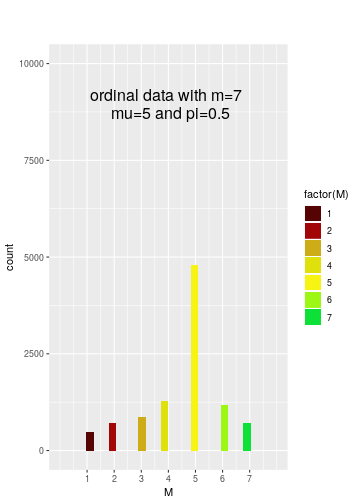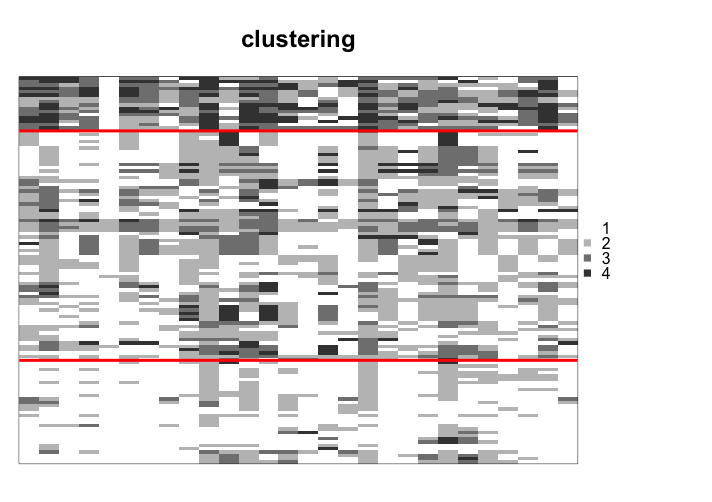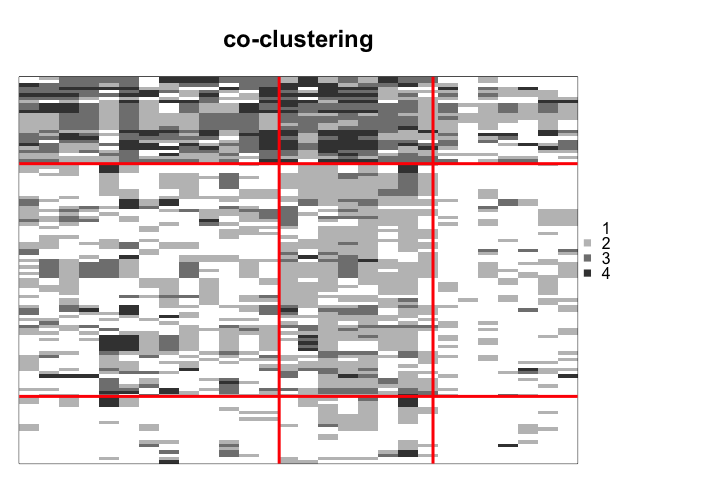Description

ordinalClust is an R package to perform classification, clustering and co-clustering of ordinal data. Furthermore, it can handle different numbers of levels and missing values. The ordinal data is considered to follow a BOS distribution [@biernacki16], which is specific to this kind of data. The Latent Block Model is used for performing co-clustering [@jacques17].

Installation

set.seed(1)
library(ordinalClust)

Datasets

The package contains real datasets created from [@Anota17]. They relate to quality of life questionnaires for patients affected by breast cancer.

• dataqol is a dataframe with 121 lines such that each line represents a patient and the columns contain information about the patient:

• Id: patient Id
• q1-q28: responses to 28 questions with the number of levels equal to 4
• q29-q30: responses to 2 questions with the number of levels equal to 7
• dataqol.classif is a dataframe with 40 lines such that a line represents a patient and the columns contain information about the patient:

• Id: patient Id
• q1-q28: responses to 28 questions with the number of levels equal to 4
• q29-q30: responses to 2 questions with the number of levels equal to 7
• death: if the patient survived (1) or not (2).

Univariate Ordinal Data Simulation

To simulate a sample of ordinal data following the BOS distribution, the function pejSim is used.

Basic example code

This snippet of code creates a sample of ordinal data with 7 levels that follows a BOS distribution parameterized by mu=5 and pi=0.5:

m=7
nr=10000
mu=5
pi=0.5

probaBOS=rep(0,m)
for (im in 1:m) probaBOS[im]=pejSim(im,m,mu,pi)
M <- sample(1:m,nr,prob = probaBOS, replace=TRUE)

Plotting

To plot the resulting distribution, the ggplot2 library can be used.Performing clustering

In this section, clustering is executed using the dataqol dataset. The purpose of performing clustering is to highlight the structure through the matrix rows.

Example code

set.seed(0)

library(ordinalClust)
data("dataqol")

M <- as.matrix(dataqol[,2:29])

m = 4

krow = 3

nbSEM=100
nbSEMburn=90
nbindmini=2
init = "randomBurnin"
percentRandomB = c(30)

object <- bosclust(x=M,kr=krow, m=m, nbSEM=nbSEM,
nbSEMburn=nbSEMburn, nbindmini=nbindmini,
percentRandomB=percentRandomB, init=init)

Plotting the result

plot(object)Performing co-clustering

Example code

In this example, co-clustering is performed using the dataqol dataset. In this case, the interest in performing co-clustering is to detect an internal structure throughout the rows and columns of the data.

set.seed(0)

library(ordinalClust)

data("dataqol")

M <- as.matrix(dataqol[,2:29])

# defining different number of categories:
m=4

# defining number of row and column clusters
krow = 3
kcol = 3

# configuration for the inference
nbSEM=100
nbSEMburn=90
nbindmini=2
init = "randomBurnin"
percentRandomB = c(30, 30)

# Co-clustering execution
object <- boscoclust(x = M,kr = krow, kc = kcol, m = m,
nbSEM = nbSEM, nbSEMburn = nbSEMburn,
nbindmini = nbindmini, init = init,
percentRandomB = percentRandomB)

Plotting the result

This snippet of code shows how to visualize the resulting co-clustering, using the plot function:

plot(object)Performing classification

In this section, the dataset dataqol.classif is used. It contains the responses to a questionnaire by 40 patients affected by breast cancer. Furthermore, a column labeled death indicates whether the patient died from the disease (2) or not (1). The aim of this section is to predict the classes of a validation dataset from a training dataset.

Choosing a good kc parameter with cross-validation

The classification function bosclassif provides two classiﬁcation models. The ﬁrst model, (chosen by the option kc=0), is a multivariate BOS model with the assumption that, conditional to the class of the observations, the features are independent. The second model is a parsimonious version of the ﬁrst model. Parsimony is introduced by grouping the features into clusters (as in co-clustering) and assuming that the features of a cluster have a common distribution. The number L of clusters of features is defined with the option kc=L. In practice, L can be chosen by cross-validation, as shown in the following example:

set.seed(1)

library(ordinalClust)
data("dataqol.classif")

M <- as.matrix(dataqol.classif[,2:29])

# creating the classes values
y <- as.vector(dataqol.classif\$death)

# sampling datasets for training and to predict
nb.sample <- ceiling(nrow(M)*7/10)
sample.train <- sample(1:nrow(M), nb.sample, replace=FALSE)

M.train <- M[sample.train,]
M.validation <- M[-sample.train,]
nb.missing.validation <- length(which(M.validation==0))

y.train <- y[sample.train]
y.validation <- y[-sample.train]

# number of classes to predict
kr <- 2

# configuration for SEM algorithm
nbSEM=200
nbSEMburn=175
nbindmini=2
init="randomBurnin"
percentRandomB = c(50, 50)

# different kc to test with cross-validation
kcol <- c(0,1,2,3)
m <- 4

# matrix that contains the predictions for all different kc
preds <- matrix(0,nrow=length(kcol),ncol=nrow(M.validation))

for(kc in 1:length(kcol)){
res <- bosclassif(x=M.train, y=y.train,
kr=kr, kc=kcol[kc], m=m,
nbSEM=nbSEM, nbSEMburn=nbSEMburn,
nbindmini=nbindmini, init=init, percentRandomB=percentRandomB)

new.prediction <- predict(res, M.validation)
preds[kc,] <- new.prediction@zr_topredict

}

preds = as.data.frame(preds)
row.names <- c()
for(kc in kcol){
name= paste0("kc=",kc)
row.names <- c(row.names,name)
}
rownames(preds)=row.names

Computing the sensitivity and specificity rates for each kc

library(caret)

actual <- y.validation -1

specificities <- rep(0,length(kcol))
sensitivities <- rep(0,length(kcol))

for(i in 1:length(kcol)){
prediction <- unlist(as.vector(preds[i,])) -1
u <- union(prediction, actual)
conf_matrix<-table(factor(prediction, u),factor(actual, u))
sensitivities[i] <- recall(conf_matrix)
specificities[i] <- specificity(conf_matrix)
}

sensitivities
##  1.0 0.5 1.0 1.0
specificities
##  0.125 0.625 0.375 0.125

Handling different numbers of levels

The package can deal with ordinal data with different numbers of levels. In this section, we show how to introduce these kinds of datasets in a co-clustering context.

Example code

In this example, co-clustering is performed using the dataset dataqol, by including the questions with 4 levels, and questions with 7 levels. The function boscoclustMulti is executed, which might take a few minutes.

set.seed(0)

library(ordinalClust)

data("dataqol")

M <- as.matrix(dataqol[,2:31])

# defining different number of categories:
m=c(4,7)

# defining number of row and column clusters
krow = 3
kcol = c(3,1)

# configuration for the inference
nbSEM=50
nbSEMburn=40
nbindmini=2
init='random'

d.list <- c(1,29)

# Co-clustering execution
object <- boscoclust(x=M,kr=krow,kc=kcol,m=m, idx_list=d.list,
nbSEM=nbSEM,nbSEMburn=nbSEMburn,
nbindmini=nbindmini, init=init)